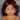# Solve Algebra Linear Equations using Backtracking

April 05, 2020

I received a picture puzzle in WhatsApp. I will explain here how I solved that using programming. I used Backtracking concept.

## Received picture puzzle

below puzzle is simple algebra concept. If you have paper and pencile then it can be solved easily. But I just wanted to use some programming concept.

## Algebra notation of the puzzle

just fill the empty circle with some letters `(a,b,c,d,e)`

Left to right

``````a  - b =  5
c  + 1 =  d
12 - e = 10``````

Top to bottom

``````a + c = 12
b - 1 =  e
5 + d = 10``````

## Program concept

now we have the equations, just take first equation `a-b=5`. This is simple equation and we can easily tell that `a and b` can have multiple posibilities like `a=10, b=5` or `a=15, b=10` etc…

we will solve the above equation using backtrack. Just asign sequence of numbers and check all the possibilites.

``````for(let a = 0; a <= 50; a++) {
for(let b = 0; b <= 50; b++) {

}
}``````

Here we created two for loops with maximum values of 50. The concept here is that loop through `a` from 0 to 50, inside that again loop through `b` from 0 to 50, which means every `a` value will be evaluated with all `b` values.

so, below is the program to solve `a-b=5`

``````for(let a = 0; a <= 50; a++) {
for(let b = 0; b <= 50; b++) {
if (a - b === 5) {
console.log(`a = \${a}, b = \${b}`)
}
}
}``````

output of the above program

``````a = 5, b = 0
a = 6, b = 1
a = 7, b = 2
a = 8, b = 3
a = 9, b = 4
...
... more values here
...
a = 47, b = 42
a = 48, b = 43
a = 49, b = 44
a = 50, b = 45``````

## Final program

we can apply the same above concept to solve the all the equations.

notation

``````a  - b =  5     ---> 1
c  + 1 =  d     ---> 2
12 - e = 10     ---> 3

a + c = 12     ---> 4
b - 1 =  e     ---> 5
5 + d = 10     ---> 6``````
``````const max = 12;

for (let a = 0; a <= max; a++) {
for (let b = 0; b <= max; b++) {
if (a - b === 5) { // <--- 1
for (let c = 0; c <= max; c++) {
if (a + c === 12) { // <--- 4
for (let d = 0; d <= max; d++) {
if (5 + d === 10 && c + 1 === d) { // <--- 6, 2
for (let e = 0; e <= max; e++) {
if (b - 1 === e && 12 - e === 10) { // <--- 5, 3
console.log(`\${a} \${b} \${c} \${d} \${e}`);
}
}
}
}
}
}
}
}
}``````

## Conclusion

Backtracking is a time consuming concept, it’s not the best solution here but let’s have fun using some program concept.Personal website and blog by M Kumaran